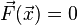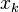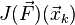Newton's method for root-finding for a vector-valued function of a vector variable

Definition

Suppose$x_1,x_2,\dots,x_n$ are variables and$F_1,F_2,\dots,F_n$ are real-valued functions of these variables, each of which is jointly differentiable with respect to the variables on a given domain. We can define the vector-valued function$\vec{F}(x_1,x_2,\dots,x_n) = (F_1(x_1,x_2,\dots,x_n),F_2(x_1,x_2,\dots,x_n),\dots,F_n(x_1,x_2,\dots,x_n))$. If we wish, we can also treat the input variables as the coordinates of a vector$\vec{x} = (x_1,x_2,\dots,x_n)$. We therefore have a differentiable$\vec{F}:\R^n \to \R^n$.

Note in particular that this Newton's method requires an equal number of variables and functions. We will discuss later in the page what we can say for cases where the number of functions is more (respectively less) than the number of variables.

The Newton's method under discussion here is a root-finding algorithm for the vector equation$\vec{F}(\vec{x}) = 0$. Note that this is a generalization of the usual Newton's method, where$n = 1$.

Iterative step

Here, we describe how to get the next guess from a given guess.

In the case of a function$f$ of one variable$x$, the next guess from a given guess$x_k$ was given as:$x_{k+1} = x_k - \frac{f(x_k)}{f'(x_k)}$

In our situation, the formula is as follows:$\vec{x}_{k+1} = \vec{x}_k - (J(\vec{F})(\vec{x}_k))^{-1}\vec{F}(\vec{x}_k)$

Here,$\vec{F}(\vec{x}_k)$ is a$n$-dimensional vector, and the Jacobian matrix$J(\vec{F})(\vec{x}_k)$ is a$n \times n$ matrix.